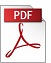### “A Finite-Element Solution of the Navier-Stokes Equations for Two-Dimensional and Axis-Symmetric Flow”

Authors: Sven Ø. Wille,
Affiliation: University of Oslo
Reference: 1980, Vol 1, No 2, pp. 105-117.

Keywords: Finite element method, blood flow, arteries

Abstract: The finite element formulation of the Navier-Stokes equations is derived for two-dimensional and axis-symmetric flow. The simple triangular, T6, isoparametric element is used. The velocities are interpolated by quadratic polynomials and the pressure is interpolated by linear polynomials. The non-linear simultaneous equations are solved iteratively by the Newton-Raphson method and the element matrix is given in the Newton-Raphson form. The finite element domain is organized in substructures and an equation solver which works on each substructure is specially designed. This equation solver needs less storage in the computer and is faster than the traditional banded equation solver. To reduce the amount of input data an automatic mesh generator is designed. The input consists of the coordinates of eight points defining each substructure with the corresponding boundary conditions. In order to interpret the results they are plotted on a calcomp plotter. Examples of plots of the velocities, the streamlines and the pressure inside a two-dimensional flow divider and an axis-symmetric expansion of a tube are shown for various Reynolds numbers.PDF (1968 Kb)        DOI: 10.4173/mic.1980.2.4

  Sven Øivind Wille (1984), doi:10.1016/0141-5425(84)90010-4  Sven Ø. Wille (1981), doi:10.4173/mic.1981.2.2  Sven Ø. Wille (1980), doi:10.1016/0307-904X(80)90184-5
References:
 HOOD, P., TAYLOR, C. (1974). Navier-Stokes equations using mixed interpolation, Proceedings of the International Symposium on Finite Element Methods in Flow Problems, pp. 121-132.
 SABERSKY, R.H., ACOSTA, A.J., HAUPTMANN, E.G. (1971). Fluid Flow, A First Course in Fluid Mechanics.Macmillan.
 TAYLOR, C., HOOD, P. (1973). A numerical solution of the Navier-Stokes equations using the finite element technique, Comput. Fluids, 1,73-100 doi:10.1016/0045-7930(73)90027-3
 ZIENKIEWICS, O.C. (1971). The Finite Element Method in Engineering Science, 2nd edition.McGraw-Hill.

BibTeX:
@article{MIC-1980-2-4,
title={{A Finite-Element Solution of the Navier-Stokes Equations for Two-Dimensional and Axis-Symmetric Flow}},
author={Wille, Sven Ø.},
journal={Modeling, Identification and Control},
volume={1},
number={2},
pages={105--117},
year={1980},
doi={10.4173/mic.1980.2.4},
publisher={Norwegian Society of Automatic Control}
};